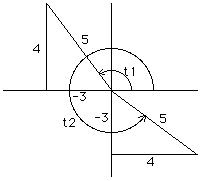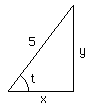```Date: Wed, 11 Dec 1996 23:09:24 -0600 (CST)
Subject: Trig problem
```
Who is asking: Student
Level: Secondary

Question:
sin t + cos t = 1/5

Find ALL exact values of cot t, given the original equation.
Please give me a hand with this, and explain it VERY well

Hi

If the fraction 1/5 makes you think of the 3-4-5 triangle then you can spot two solutions.In the second quadrant the angle t1 has sin t1 = 4/5 and cos t1 = -3/5 so cot t1 = -3/4. In the fourth quadrant the angle t2 has sin t2 = -3/5 and cos t2 = 4/5 so cot t2 = -4/3.
Another approach, and one that shows the angles above are the only angles between 0 and 360 degrees that solve your problem, is to consider a right triangle with on angle t and sides of length x and y as in the diagram. The hypotenuse then has length sqrt(x^2 + y^2). Since the trig functions depend only on ratios of the lengths of the sides I can take the triangle to be of any size, so take the hypotenuse to have length 5, that is x^2 + y^2 = 25.Thus if t is an angle that solves your problem then x/5 + y/5 = 1/5 and x^2 + y^2 = 25. Since the graph of the first equation is a straight line and the graph of the second equation is a circle there can be at most two points of intersection. Alternatively you can solve the two equations. The first gives y = 1 - x. Substituting into the second and simplifying gives x^2 - x - 12 = 0 or (x-4)(x+3)=0. Thus x = -3 or x = 4 giving the 3-4-5 triangle mentioned above.
Cheers
Harley

Go to Math Central

To return to the previous page use your browser's back button.